Using a Graphics Calculator to Perform Matrix Operations The KML students creating the Mathman clues have the advantage of having graphic calculators.  These calculators make certain operations with matrices MUCH easier to perform than calculating them by hand. Adding or subtracting matrices is easier and faster to do by hand.  All you have to do is add/subtract the corresponding numbers in the matrices and place the result in the same position in the resulting matrix.  Scalar multiplication is also simple to do by hand.  All you need to do is multiply the one number (the scalar) times each of the numbers in the matrix one at a time, and write the resulting numbers in the same position in the resulting matrix. Matrix multiplication and finding/using inverses of matrices are much more tricky.  Although students are taught how to do this by hand, due to the amount of time and numerous places for making mistakes involved in working through these operations, using some kind of computing device is generally recommended in actual practice. Since you probably do not have a graphics calculator, and they are expensive, here is a free graphic calculator simulator you can use.  Follow the directions on the webpage to install it on your computer.  It does everything the regular calculator does except for the portability issue.  (It also clears its memory every time the program is exited.)  I would suggest loading it onto a laptop for use out in the letterboxing field.  If you only have a desktop computer, you'll have to figure out a method that works for you (or see if you can borrow a graphics calculator from a teenager or math teacher). (You can try to do these by hand.  Use a regular calculator to do matrix multiplication (scroll to "matrix multiplication as combination of rows" (page 8) for an easy method) and inverses of matrices (scroll to page 2 for a 2x2 shortcut).  Again, this part of matrix mathematics is one of the more time consuming, confusing, and difficult parts of high school mathematics to learn and do without a graphics calculator (but also one of the most applicable).) If you are using the simulator or have borrowed a TI-83/84 type graphics calculator, use the following tutorial to learn how to work with matrices on this type of calculator. Exploring Matrix Operations with the TI-83+ (Adapted from Betty Martin Gasque, 2001) To enter matrix values in the calculator, press the MATRIX key (the 2nd shift of the x-1 key).  Move to the right (with the blue right arrow key) to the EDIT column.  Press the ENTER key or the number 1 key to edit matrix [A].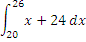First, enter the dimensions of the matrix giving the number of rows first and the number of columns second.  Press the ENTER key after entering each number.  The matrix array shown below is a 2 by 3 (2 x 3) matrix.  (When entering negative values in the calculator, be sure to use the negative key (-) on the bottom row of the calculator and not the subtraction key.)  After the last value is entered in matrix [A] (be sure you hit ENTER after typing the last number so it changes on the screen), press the 2nd key followed by the MODE key to QUIT the matrix.  To enter values in other matrices, repeat this process using [B], [C], etc.   [3         4          -2                                 0.5       -1         6.2]Look at the matrices below and determine the dimension of each matrix.  Give the dimension of each.  Give the number of rows first and the number of columns second.   [B]  2 x 2         [C]  ____         [D]  ____         [E]  ____         [F]  ____   Enter the values shown below in matrices [B] through [F] in the MATRIX EDIT location of the calculator.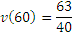To add, subtract, or multiply matrices, go to the home screen of the calculator.  (Press the 2nd key followed by the MODE key to return to the home screen if needed. You may need to press the CLEAR key to clear off the home screen.)  If you get a “DIM MISMATCH” error with any operation that means the dimensions of the matrices involved are not appropriate for the operation involved.  For example, to add two matrices together they must have the exact same dimensions, so one could not add [A] and [B]..   To add matrices [B] and [E], press the MATRIX key (2nd  x-1) and press the number 2 key for the name of matrix [B].  Press the addition (+) key and then press the MATRIX (2nd  x-1) key again and choose number 5 for matrix [E].  Press ENTER.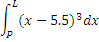To multiply matrices [E] and [A], press the MATRIX key (2nd  x-1) and press the number 5 key for the name of matrix [E].  Press the multiplication (*) key (optional – the calc knows implied multiplication) and then press the MATRIX (2nd  x-1) key again and choose number 1 for matrix [A].  Press ENTER.Now let’s work with matrices to code/decode clues.  For information on how this is done go here. (Note: We use 0 = 'space' not 27, otherwise 1='A' and 26='Z'.)  Usually the matrices we use have 2 rows and 2 columns, so the coding/decoding matrix is a 2x2, and the four digit numbers you find make up the four numbers of the 2x2 coding matrix, not the decoding matrix (which is the inverse of the coding matrix).  For practice, try decoding the following message (which uses the 4 digit number 5342 for the 2x2 coding/decoding matrix).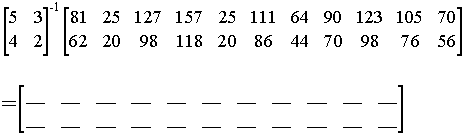Enter the first matrix into matrix [A] as a 2x2 and enter the second matrix into matrix [B] as a 2x11.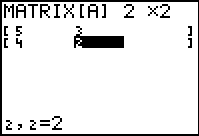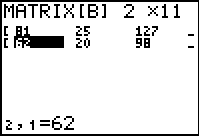To multiply matrices [A]-1 and [B], press the MATRIX key (2nd  x-1) and press the number 1 key for the name of matrix [A].  Press the [x-1] key to get the raised -1 (this tells the calculator to use the inverse of matrix [A]). Press the MATRIX (2nd  x-1) key again and choose number 2 for matrix [B].  Press ENTER.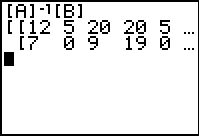Now copy the resulting numbers into the blank matrix. The “…” on the calculator screen means you can press the blue right arrow to scroll for the rest of the matrix off the screen (left would scroll left). Once you have all the numbers written down, use the following key to decode the message: KEY: SPACE=0  A=1  B=2  C=3  D=4  E=5  F=6  G=7  H=8  I=9  J=10  K=11  L=12  M=13  N=14  O=15  P=16  Q=17  R=18  S=19  T=20  U=21  V=22  W=23  X=24  Y=25  Z=26 You should get the message "LETTERBOXING_IS_GREAT_". (Side note: Multiplying [A] by the resulting matrix would produce [B].  This is how the students encoded the message in the first place.) Some of the Mathman clues just have you add/subtract matrices to get the matrix to decode instead of using the matrix inverse method.  Some others use larger encoding/decoding matrices.  Hopefully now you’ll feel more confident to give these a try!